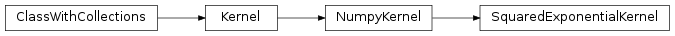# mvpa2.clfs.warehouse.SquaredExponentialKernel¶class `mvpa2.clfs.warehouse.``SquaredExponentialKernel`(length_scale=1.0, sigma_f=1.0, **kwargs)

The Squared Exponential kernel class.

Note that it can handle a length scale for each dimension for Automtic Relevance Determination.

Attributes

 `descr` Description of the object if any `length_scale`

Methods

 `add_conversion`(typename, methodfull, methodraw) Adds methods to the Kernel class for new conversions `as_ls`(kernel) `as_np`() Converts this kernel to a Numpy-based representation `as_raw_ls`(kernel) `as_raw_np`() Directly return this kernel as a numpy array. `as_raw_sg`(kernel) Converts directly to a Shogun kernel `as_sg`(kernel) Converts this kernel to a Shogun-based representation `cleanup`() Wipe out internal representation `compute`(ds1[, ds2]) Generic computation of any kernel `compute_lml_gradient`(alphaalphaT_Kinv, data) Compute grandient of the kernel and return the portion of log marginal likelihood gradient due to the kernel. `compute_lml_gradient_logscale`(...) Compute grandient of the kernel and return the portion of log marginal likelihood gradient due to the kernel. `computed`(\*args, \*\*kwargs) Compute kernel and return self `reset`() `set_hyperparameters`(hyperparameter) Set hyperaparmeters from a vector.

Initialize a Squared Exponential kernel instance.

Parameters: length_scale : float or numpy.ndarray, optional the characteristic length-scale (or length-scales) of the phenomenon under investigation. (Defaults to 1.0) sigma_f : float, optional Signal standard deviation. (Defaults to 1.0) enable_ca : None or list of str Names of the conditional attributes which should be enabled in addition to the default ones disable_ca : None or list of str Names of the conditional attributes which should be disabled

Attributes

 `descr` Description of the object if any `length_scale`

Methods

 `add_conversion`(typename, methodfull, methodraw) Adds methods to the Kernel class for new conversions `as_ls`(kernel) `as_np`() Converts this kernel to a Numpy-based representation `as_raw_ls`(kernel) `as_raw_np`() Directly return this kernel as a numpy array. `as_raw_sg`(kernel) Converts directly to a Shogun kernel `as_sg`(kernel) Converts this kernel to a Shogun-based representation `cleanup`() Wipe out internal representation `compute`(ds1[, ds2]) Generic computation of any kernel `compute_lml_gradient`(alphaalphaT_Kinv, data) Compute grandient of the kernel and return the portion of log marginal likelihood gradient due to the kernel. `compute_lml_gradient_logscale`(...) Compute grandient of the kernel and return the portion of log marginal likelihood gradient due to the kernel. `computed`(\*args, \*\*kwargs) Compute kernel and return self `reset`() `set_hyperparameters`(hyperparameter) Set hyperaparmeters from a vector.
`compute_lml_gradient`(alphaalphaT_Kinv, data)

Compute grandient of the kernel and return the portion of log marginal likelihood gradient due to the kernel. Shorter formula. Allows vector of lengthscales (ARD).

`compute_lml_gradient_logscale`(alphaalphaT_Kinv, data)

Compute grandient of the kernel and return the portion of log marginal likelihood gradient due to the kernel. Hyperparameters are in log scale which is sometimes more stable. Shorter formula. Allows vector of lengthscales (ARD).

`length_scale`
`reset`()
`set_hyperparameters`(hyperparameter)

Set hyperaparmeters from a vector.

Used by model selection.